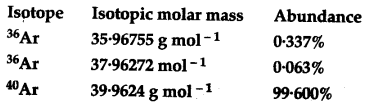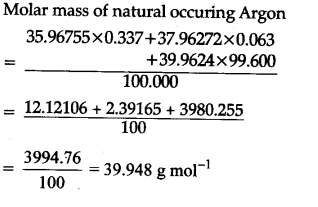# Use the data given in the following table to calculate the molar mass of naturally occurring argon isotopes:

Use the data given in the following table to calculate the molar mass of naturally occurring argon isotopes: